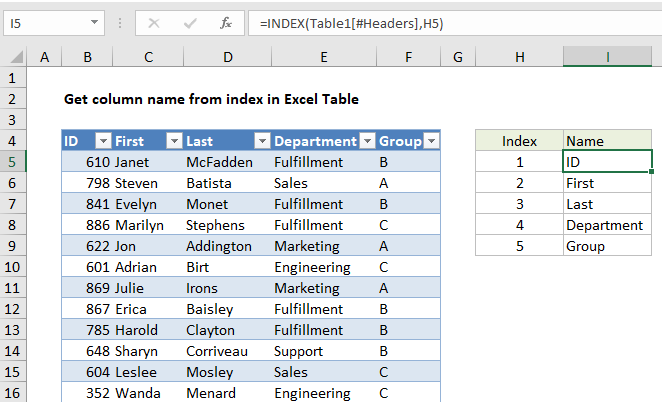## Excel Office

Excel How Tos, Tutorials, Tips & Tricks, Shortcuts

# Get column name from index in Excel Table

To get the name of a column in an Excel Table from its numeric index, you can use the INDEX function with a structured reference. See example below:

## Formula

`=INDEX(Table[#Headers],index)`## Explanation

In the example shown, the formula in I4 is:

`=INDEX(Table1[#Headers],H5)`

When the formula is copied down, it returns an name for each column, based on index values in column H.

Worked Example:   Calculate Conditional Percentile 'IF' in table in Excel

### How this formula works

This is a standard INDEX formula. The only trick to the formula is the use of a structured reference to return a range for the table headers:

`Table1[#Headers]`

This range goes into INDEX for the array argument, with the index value supplied from column H:

`=INDEX(Table1[#Headers],H5)`

The result is he name of the first item in the header, which is “ID”.

Worked Example:   How to count table rows in Excel

Although the headers are in a horizontal array, with values in columns, INDEX will use the row number as a generic INDEX for one-dimensional arrays like this and correctly return the value at that position.